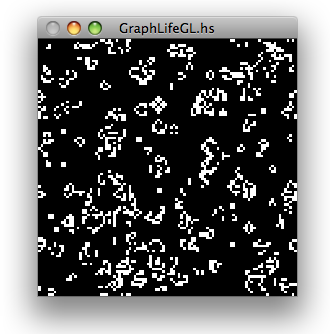# GraphLifeThis is a somewhat odd cellular automaton implementation in Haskell. It encodes the shape of the world, and the stepping rule, in the data structure. Briefly:

```data Cell v n = Cell { value     :: v,
neighbors :: n (Cell v n),
future    :: Cell v n }

```
```-- Build a cell and its chain of futures, given its rule, similar neighbors,
-- and initial value.
mkCell :: Functor n => (v -> n v -> v) -> v -> n (Cell v n) -> Cell v n
mkCell rule val nei = initial
where initial = Cell val nei (calcFuture initial)
calcFuture c = c'
where c' = Cell (rule (value c) (fmap value (neighbors c)))
(fmap future (neighbors c))
(calcFuture c')

data Moore c = Moore c c c c c c c c

-- Conway's Life
evalLife :: Bool -> Moore Bool -> Bool
evalLife v n = f v (sumN fromEnum n)
where f _    3 = True
f True 2 = True
f _    _ = False```

The remainder of the code is routines for setting up worlds and displaying them.